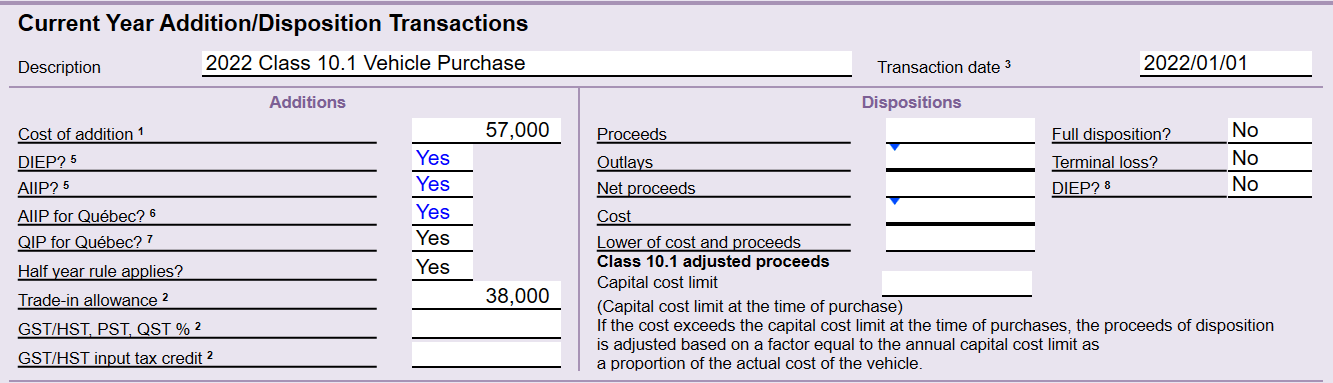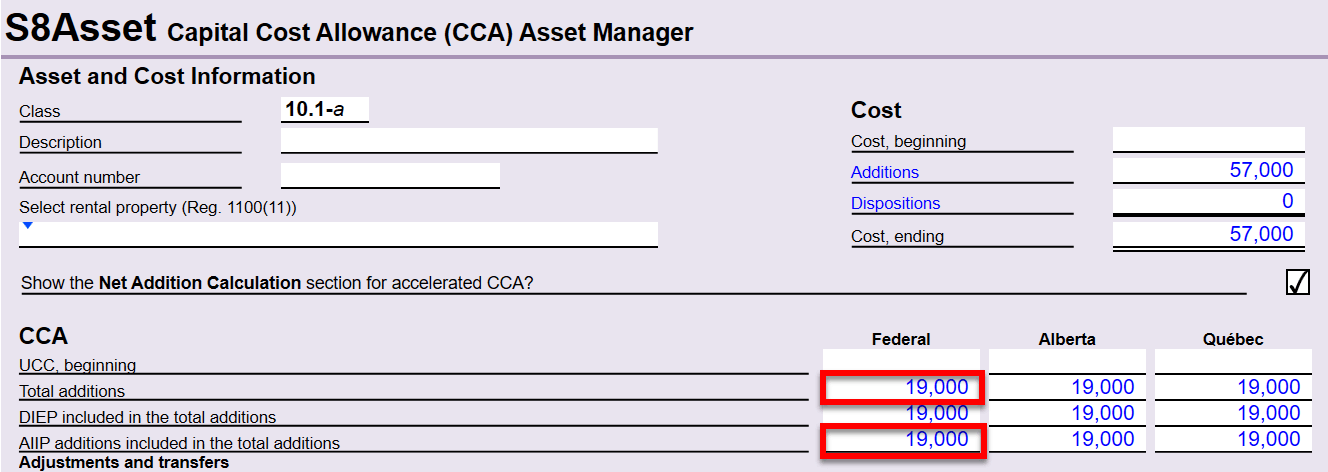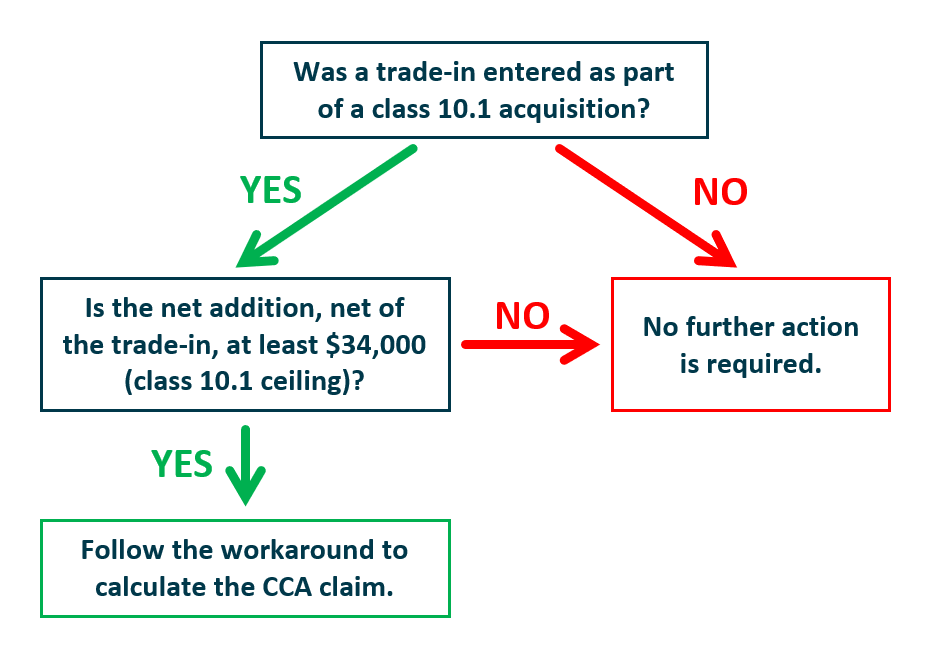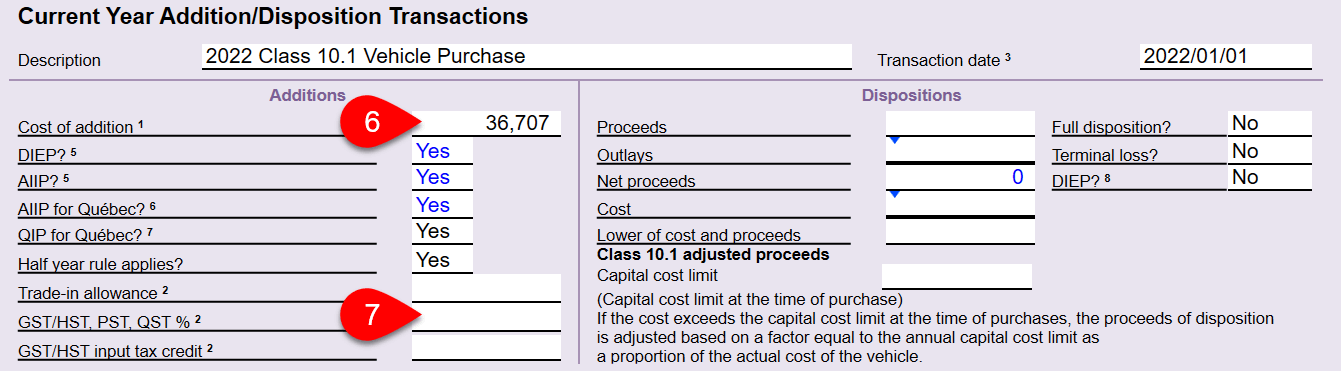When you enter a trade-in allowance on the S8Asset, TaxCycle incorrectly reduces the class 10.1 capital cost by the amount of the trade-in.

#### Example

Price of class 10.1 vehicles: \$57,000*
Purchase date: January 1, 2022

 Purchase price paid in cash \$19,000 Trade in (payment in kind) \$38,000 Total payment \$57,000

* 2022 class 10.1 ceiling amount = \$34,000TaxCycle understates the capital cost as \$19,000, which also causes an understated CCA claim.The correct class 10.1 capital cost is \$34,000, calculated as the lower of the gross purchase price of \$57,000 and the 2022 class 10.1 ceiling amount of \$34,000. The vehicle was paid for in cash and in kind. The trade-in is considered a payment in kind and must NOT reduce the capital cost.

Note: If a class 10.1 acquisition does not involve a trade-in, or if the net capital cost after the trade-in is equal to or greater than the class 10.1 ceiling amount (the 2022 class 10.1 ceiling is \$34,000), TaxCycle correctly calculates the capital cost amount.

In the example, the capital cost amount net of trade-in is \$19,000 (\$57,000 minus \$38,000) and is less than the 2022 class 10.1 ceiling of \$34,000. Therefore, the calculated class 10.1 capital cost is incorrect.

Alternatively, if the trade-in amount in this example was much lower, such as \$7,000, the capital cost amount net of trade-in would be \$50,000 (\$57,000 minus \$7,000), and is at least the class 10.1 ceiling amount or above. Therefore, TaxCycle correctly calculates the class 10.1 addition amount.

## Decision Chart

Use the following chart to decide whether to use the workaround when claiming class 10.1.## Workaround Example

First, calculate the tax to be capitalized as part of the class 10.1 capital cost:

1. Calculate the capital cost amount by subtracting the trade-in value from cost of addition.
\$67,383 - \$44,822 = \$22,561
2. To determine the net capital cost, calculate the lower of the following two amounts:
• Net capital cost amount from step 1: \$22,561
• Class 10.1 ceiling amount: \$34,000
3. Apply the tax rate to the amount from step 2.
\$22,561 × 12% = \$2,707

Next, calculate the correct capital cost:

1. Add the class 10.1 ceiling and the tax from step 3.
\$34,000 + \$2,707 = \$36,707
2. If applicable, subtract any GST/HST input tax credit amount from the capital cost calculated in step 4. This is the final capital cost amount to use for class 10.1 acquisition.
\$36,707 - \$0 = \$36,707

Finally, enter the addition on the S8Asset:

1. Enter the amount from step 5 in the Cost of addition field.
2. Remove the amounts from the Trade-in allowance, GST/HST, PST, QST % and GST/HST input tax credit fields.## Resolution

This issue was resolved in the latest TaxCycle update.